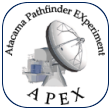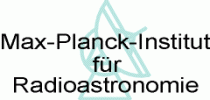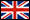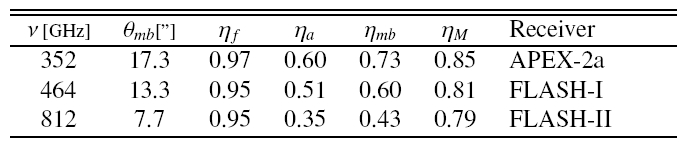Atacama Pathfinder EXperiment APEX TelescopeAPEX TWIKI CONTACT SEARCH HELP NEWS DISCLAIMER# APEX Antenna Efficiencies

20162017201820192020

MarsJupiterUranusAll

Instrument Year-Month Planet θS ('')1 Frequency (GHz) θmb ('') ηa2,4 ηmb3,4 Jy/K5 # Scans
NFLASH2302020-01MARS4.7 ± 0.00230.7126.20.68 ± 0.060.83 ± 0.0735 ± 36

Note 1: The planet sizes (θS) and fluxes from the planet models usually have < 5% uncertanties. The values given in the table above are the average planet size from selected scans (at least 5 scans at a given frequency). If the planet size is smaller than 0.4 x HPBW (θmb) then θS, aperture efficiency (ηa) and the point source sensitivity (Jy/K) will be highlighted since the correction [1-exp(-Q)]/Q (with Q=ln(2)(θSmb)²) for non-pointlike source is < 5%.

Note 2: The aperture efficiency (ηa) intrinsicly includes the effects due to error beams, blockage, spillover, etc., because it is measured on sky toward a planet. Thus, it is lower than the expected aperture efficiency due exclusively to the surface accuracy as described by the original Ruze equation.

Note 3: Although the correction for the influence of the source size mentioned in Note 1 is only a first order correction (i.e, it asumes a clean Gaussian beam and does not take into account the effect of the sidelobes nor the error beams), we can consider our estimates a good approximation of the actual main beam efficiencymb). The spectra delivered by the APEX real-time calibration software (Muders et al. 2006, A&A, 454, L25) is in GILDAS/CLASS format (Pety J., 2005, in SF2A-2005, p. 721) in the corrected antenna temperature TA* scale (Ulich & Hass, 1976, ApJ, Suppl. Ser., Vol. 30, p. 247) with ηf=0.95 and ηmb=1 in the headers. Since CLASS automatically applies the conversion factor ηf / ηmb to the spectra, one should use the following sequence of commands:

get [obs.number]
modify beam_eff 0.95
pen 0; plot;
(this shows the CLASS variable RY = TA* from APEX, since ηf / ηmb = 1)

modify beam_eff [value from table]
pen 1; spec;
(this shows the spectrum RY = Tmb scale)

For point-like (relative to the beam size) science targets we recommend to use the ηmb values estimated from observations towards Uranus or Mars (when smaller than 0.4 x HPBW). For semi-extended sources (not point-like but unresolved by the beam) values estimated from Mars (when 0.4 x HPBW < θS < HPBW) are better. For extended sources (resolved by the beam) the values estimated from Jupiter or Mars (when larger than the beam) are recommended.

Note 4: Because of the new sub-reflector the forward efficiency (ηf=0.95) for 2018+ have smaller uncertainty of about 5% in contrast to the 10% uncertainty considered for previous years. Other efficiencies (ηa, ηmb and Jy/K) include the uncertainties of: the forward efficiency, the planet model (4%), the beam size (5%) and the uncertainty of the observed peak brightness temperature (usually < 10% for Uranus, and < 5% for Mars and Jupiter).

Note 5: The Jansky to Kelvin conversion factor for the APEX antenna can also be approximated by ηf24.4/ηa (Jy/K). According to the Ruze formula the aperture efficiency (ηa) and, hence, the main beam efficiency (ηmb), will degrade with shorter wavelength, λ, as

ηa (λ) = ηa (∞) exp(-16π²σ²/λ²)

where σ is the antenna surface RMS value (i.e. describing small scale deviations from a perfect surface).

Likewise, according to Downes (1989) the main beam efficiency can be estimated from the aperture efficiency as

ηmb (λ) = 0.8899 [θmb/(λ/D)]² ηa

with θmbk λ/D (assuming a Gaussian beam) where k is a factor that depends on the illumination taper and blockage of the aperture (e.g., Christiansen & Högbom 1985; Kraus 1986). For most APEX receivers we found k ≈ 1.17 which correspond to an edge tapering of about 13.9 dB (consistent with the value assumed by Güsten et al. 2006). Thus, the main beam efficiency can be approximated as: ηmb (λ) = 0.8899 (1.17)² ηa.

From the 2018 commissioning we recommend using θmb ≈ (9.0 ± 0.1) 658/fGHz for the full frequency range of SEPIA660.

For the estimates we select only well focused and calibrated pointing scans toward Mars, Jupiter and Uranus (when available; during most of 2017 Mars was in sun avoidance). Pointing scans with offsets smaller than 10% of the beam size are selected for the estimates. Only the cross-scan in Azimuth are used and we discard the scans where the FWHM from the Azimuth and Elevation cross-scans differ by more than 10%. The aparent size and fluxes of the planets for a particular date and frequency are obtained from the corresponding models implemented in CASA ( CASA Guides: Solar System Models; ALMA memo #594). Comparing with other existing models (e.g., in GILDAS/ASTRO and in the work by Lellouch et al., 2006) we found they agree within 15%. Details of the theory and calculations used in our estimates can be found here. For a more elaborated explanation of the formulas we refer to the IRAM 30m internal documents cali_rep.pdf or spatial_response_framework_v1.8.pdf.

Efficiencies for the PI CHAMP+ instrument can be found at the corresponding web page at MPIfR.

The historical table below was adapted from Güsten et al. (2006, A&A 454, L13-L16). Beam sizes (θmb) were determined by deconvolving planet scans. The listed efficiencies are forward efficiency (ηf), aperture efficiency (ηa), main beam efficiency (ηmb), and Moon efficiency (ηM). The uncertainties of the listed efficiencies are expected to be 5-10%. For comparison with older values you can still find the former table of antenna efficiencies here.